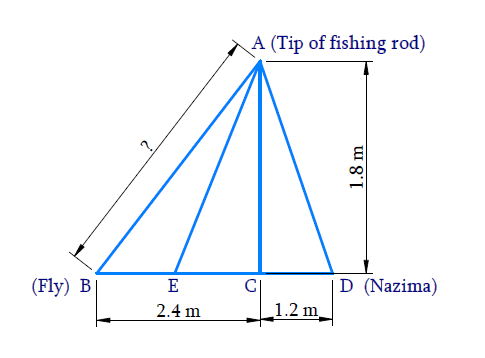In the verge of coronavirus pandemic, we are providing FREE access to our entire Online Curriculum to ensure Learning Doesn't STOP!

# Ex. 6.6 Q10 Triangles Solution - NCERT Maths Class 10

Go back to  'Ex.6.6'

## Question

Nazima is fly fishing in a stream. The tip of her fishing rod is $$1.8\,\rm m$$ above the surface of the water and the fly at the end of the string rests on the water $$3.6\,\rm m$$ away and $$2.4\,\rm m$$ from a point directly under the tip of the rod. Assuming that her string (from the tip of her rod to the fly) is taut, how much string does she have out (see Fig. )? If she pulls in the string at the rate of $$5\,\rm cm$$ per second, what will be the horizontal distance of the fly from her after $$12\,\rm seconds$$?

####Video Solution
Triangles
Ex 6.6 | Question 10

## Text Solution

#### Reasoning:

Pythagoras Theorem

#### Steps:

To find $$AB$$ and $$ED$$

$$BD = 3.6\, {\rm m} ,\\ BC = 2.4 \,{\rm m} , \\ CD = 1.2\,{\rm m},\, \\ AC = 1.8\,\rm cm$$

In $$\Delta ACB$$

\begin{align} A{B^2} &= A{C^2} + B{C^2}\\ &= {\left( {1.8} \right)^2} + {\left( {2.4} \right)^2}\\ &= 3.24 + 5.76\\ A{B^2} &= 9.00\\AB &= 3 \end{align}

Length of the string out $$AB = \rm{3\,cm}$$

Let the fly at $$E$$ after $$12\,\rm{seconds}$$

String pulled in $$12\,\rm{ seconds}$$

\begin{align} &= 12 \times 5\\&= 60 \rm{cm}\\ &= 0.6 \rm{m}\\ AE &= 3\,\rm{m}-0.6\, \rm{m}\\ &= 2.4 \,\rm{m} \end{align}

Now in $$\Delta ACE$$

\begin{align} C{E^2}& = A{E^2} - A{C^2}\\ &= {\left( {2.4} \right)^2} - {\left( {1.8} \right)^2}\\ C{E^2} &= 5.76 - 3.24\\ &= 2.52\\ CE& = 1.587\;\rm{}m \end{align}

\begin{align} DE &= CE + CD\\ &= 1.587 + 1.2\\ &= 2.787\\ DE& = 2.79\;\rm{}m \end{align}

Horizontal distance of the fly after $$12\,\rm seconds$$ $$= 2.79\, \rm m$$

Learn from the best math teachers and top your exams

• Live one on one classroom and doubt clearing
• Practice worksheets in and after class for conceptual clarity
• Personalized curriculum to keep up with school